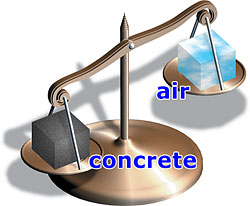# DENSITY OF CONCRETE

## WHAT IS THE DENSITY OF CONCRETE?

The density of concrete is a measure of its unit weight. Concrete is a mixture of cement, fine and coarse aggregates, water, and sometimes some supplementary materials like fly ash, slag, and various admixtures.A normal weight concrete weighs 2400 kg per cubic meter or 145 lbs per cubic foot (3915 lbs per cubic yard).

The unit weight of concrete (density) varies depending on the amount and density of the aggregate, the amount of entrained air (and entrapped air), and the water and cement content.

### Concrete Weight - How Much Does Concrete Weigh?

The concrete weight can vary depending on the air and moisture content it contains. Cement density (weight) can be 830 - 1650 kg per cubic meter or 52 - 103 lbs per cubic foot.

Cement that is pneumatically loaded into silos is less dense while cement that has been stored and exposed to vibration, like being transported, is more dense. The rule of thumb is to consider that a 94 lb. bag of cement will make one cubic foot when it is freshly packed.

The density of lightweight concrete is 1920 kg per cubic meter or 116 lbs per cubic foot (3132 lbs per cubic yard). Lightweight concrete weighs less because it is made with an aggregate called pumice, a naturally light mineral.

Density is simply a mass to volume ratio. Perhaps the easiest and most accurate way to calculate the concrete density is to measure some into a container of known volume and weighing it.

One value of understanding, knowing, and monitoring the density of concrete is checking strength by using test cylinders. A reduced density of normal concrete almost always means a higher water content which means lower strength concrete.

These strength tests can be done at 24 hrs, 7 days, and 28 days in a lab to predict possible strength downturns (or lower density). This is very important considering all the high strength projects (bridges, high rises, dams) concrete is used for.

For people considering concrete counter tops or interior radiant floor heating, you may be wondering if using lightweight concrete would help to minimize stress on the cabinetry and flooring below.

With a standard thickness of 1.5 inches, normal weight concrete weighs about 18 lbs per square foot while lightweight concrete weighs 14.5 lbs per square foot.

The 3.5 lbs per square foot weight savings is not usually enough to make a significant difference with concrete counter tops and normal density concrete is easier to polish.

For flooring, a large surface area would result in a considerable concrete weight savings and would be worth considering. Keep in mind lightweight concrete is usually double the price of regular concrete.

## How to calculate how much a square foot of concrete weighs

The weight of a square foot of concrete will depend on how THICK that square foot of concrete is.

Here is a simple calculation to figure the weight of a square foot of concrete based on how thick the concrete is.

A CUBIC FOOT OF CONCRETE WEIGHS 145 POUNDS

1. Divide the thickness of your concrete by 12 (this gives you the depth in feet.)

For example: For a 6 inch slab of concrete - divide 6 by 12 and you get .5  (a 6 inch slab is .5 feet thick)

2. Multiply 145 (pounds of a cubic foot) by .5 (thickness in feet) and you get 72.5 pounds.

Conclusion: A 6 inch thick slab weighs 72.5 pounds per square foot.

HOW MUCH DOES A 4" THICK SLAB OF CONCRETE WEIGH?

4 / 12 = .33

145 X .33 = 47.85 POUNDS PER SQUARE FOOT

If you need to accurately measure the temperature of your concrete, this infrared digital laser thermometer is the same one I use.

You can get it right from Amazon.

Disclaimer: I am an affiliate of Amazon.com, If you use my link to purchase anything within 24 hours from Amazon, this site will make a small commission. Thank you for your support of this site!

Understanding concrete density is an important part of knowing the possibilities and limitations of what concrete can be used for.

For other interesting facts about concrete read below:

PROPERTIES OF CONCRETE

FLY ASH CONCRETE

SCC CONCRETE ALSO KNOW AS SELF-CONSOLIDATING CONCRETE

LIGHTWEIGHT CONCRETE

REDI MIX CONCRETE

WHAT IS SHOTCRETE?

Return from Density of concrete to History of concrete

One more way to share everything-about-concrete.com